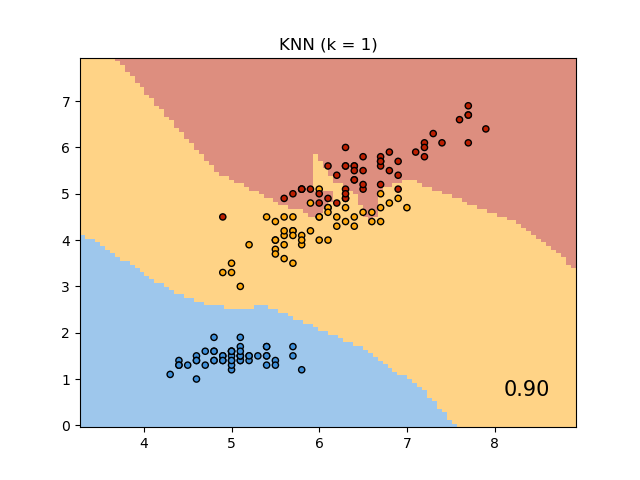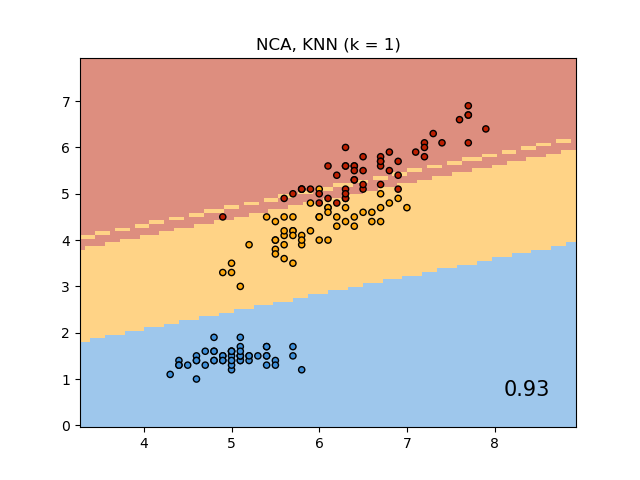# Comparing Nearest Neighbors with and without Neighborhood Components Analysis¶

An example comparing nearest neighbors classification with and without Neighborhood Components Analysis.

It will plot the class decision boundaries given by a Nearest Neighbors classifier when using the Euclidean distance on the original features, versus using the Euclidean distance after the transformation learned by Neighborhood Components Analysis. The latter aims to find a linear transformation that maximises the (stochastic) nearest neighbor classification accuracy on the training set.

••# License: BSD 3 clause

import matplotlib.pyplot as plt
from matplotlib.colors import ListedColormap
from sklearn import datasets
from sklearn.model_selection import train_test_split
from sklearn.preprocessing import StandardScaler
from sklearn.neighbors import KNeighborsClassifier, NeighborhoodComponentsAnalysis
from sklearn.pipeline import Pipeline
from sklearn.inspection import DecisionBoundaryDisplay

n_neighbors = 1

X, y = dataset.data, dataset.target

# we only take two features. We could avoid this ugly
# slicing by using a two-dim dataset
X = X[:, [0, 2]]

X_train, X_test, y_train, y_test = train_test_split(
X, y, stratify=y, test_size=0.7, random_state=42
)

h = 0.05  # step size in the mesh

# Create color maps
cmap_light = ListedColormap(["#FFAAAA", "#AAFFAA", "#AAAAFF"])
cmap_bold = ListedColormap(["#FF0000", "#00FF00", "#0000FF"])

names = ["KNN", "NCA, KNN"]

classifiers = [
Pipeline(
[
("scaler", StandardScaler()),
("knn", KNeighborsClassifier(n_neighbors=n_neighbors)),
]
),
Pipeline(
[
("scaler", StandardScaler()),
("nca", NeighborhoodComponentsAnalysis()),
("knn", KNeighborsClassifier(n_neighbors=n_neighbors)),
]
),
]

for name, clf in zip(names, classifiers):

clf.fit(X_train, y_train)
score = clf.score(X_test, y_test)

_, ax = plt.subplots()
DecisionBoundaryDisplay.from_estimator(
clf,
X,
cmap=cmap_light,
alpha=0.8,
ax=ax,
response_method="predict",
plot_method="pcolormesh",
)

# Plot also the training and testing points
plt.scatter(X[:, 0], X[:, 1], c=y, cmap=cmap_bold, edgecolor="k", s=20)
plt.title("{} (k = {})".format(name, n_neighbors))
plt.text(
0.9,
0.1,
"{:.2f}".format(score),
size=15,
ha="center",
va="center",
transform=plt.gca().transAxes,
)

plt.show()


Total running time of the script: ( 0 minutes 0.460 seconds)

Gallery generated by Sphinx-Gallery